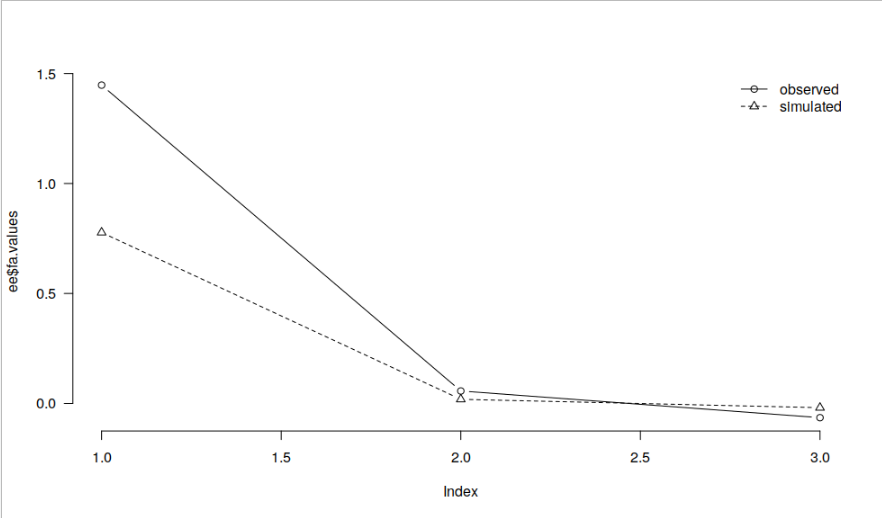#### Howdy, Stranger!

It looks like you're new here. If you want to get involved, click one of these buttons!

Supported by

# Parallel Analysis in JASP shows persistent discrepancy with R across samples and items

Hello,

I am adding this as a new discussion, as there were not any responses to the older discussion on this that I followed up upon (https://forum.cogsci.nl/discussion/5382/parallel-analysis-for-efa-in-jasp#latest)

I have the same issue as described in the discussion above: JASP always suggests a different number of factors compared to R in parallel analysis, irrespective of which fa.parallel() settings I use, i.e., SMC = TRUE does not make a difference. I have tried this for 3 different combinations of questionnaires (different combinations of items from the same data set in this case), and I have repeated the parallel analysis several times.

Could there maybe be a different explanation for why this happens, other than that the difference is due to chance (because the simulated data can be a little different every time) as pointed out by @evankesteren? The factor loadings are identical as expected when I use "minres" for both. And it seems odd to me that there is a consistent considerable difference due to chance.

As an example, R suggests 3 whereas JASP suggests 1 factor here. I can see how both make sense, but this happens for any combination of items.

Any help is appreciated!

• I asked Erik-Jan (who has just started as assistant professor in Utrecht) and he mentions this is somewhat of a puzzle. "Someone" should study the parallel analysis method with help of the data presented. Let me see if I can find "someone"...

Cheers,

E.J.

• Could you perhaps share the code and/ or data you use so that we can replicate this discrepancy? In the simple example below, I get basically identical results in JASP and R.

```dd <- read.csv("https://raw.githubusercontent.com/jasp-stats/jasp-desktop/development/Resources/Data%20Sets/debug.csv")
ee <- psych::fa.parallel(dd[, c("contNormal", "contcor1", "contcor2")], fa = "fa", plot = FALSE)

par(las = 1, bty = "n")
plot(ee\$fa.values, type = "b")
lines(ee\$fa.sim, type = "b", lty = 2, pch = 2)
legend("topright", legend = c("observed", "simulated"), lty = 1:2, pch = 1:2, col = 1, bty = "n")```

and JASP:

• Thank you for getting back to me.

The scree plots are indeed identical in both examples in my data, too, so are the item factor loadings, but the number of factors suggested by parallel analysis differ.

JASP:

R:

Scree plot in my initial post (looks the same as the JASP one I think)

The recommendation for 3 factors does not change based on whether SMC is TRUE or FALSE, or whether I add the cor = "poly" argument (though I do get a warning then, which is understandable).

A model with 1 factor has a worse fit than a model with 2 factors. A model with 3 factors has a better fit but the latter two factors are too small, so I settled for the model with 2 factors in this pilot study which has a pretty good fit already (RMSEA = .062; TLI = 0.954).

As I have now collected the data from the second study I'd like to make sure I use the right method for parallel analysis.

If it still helps if I were to send the anonymised data please let me know and I will do so (via a private message / email).